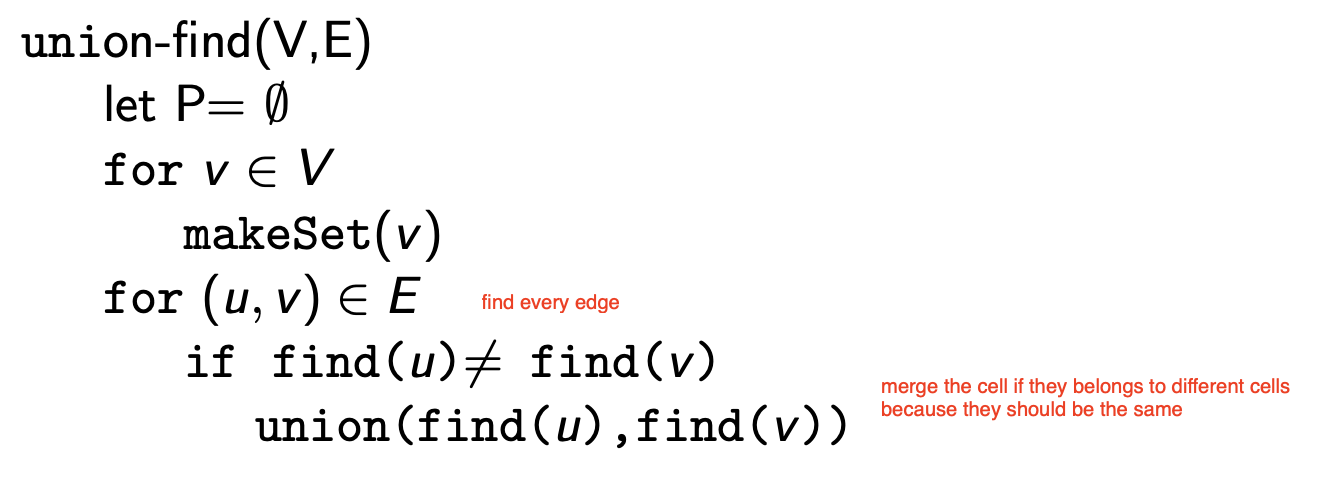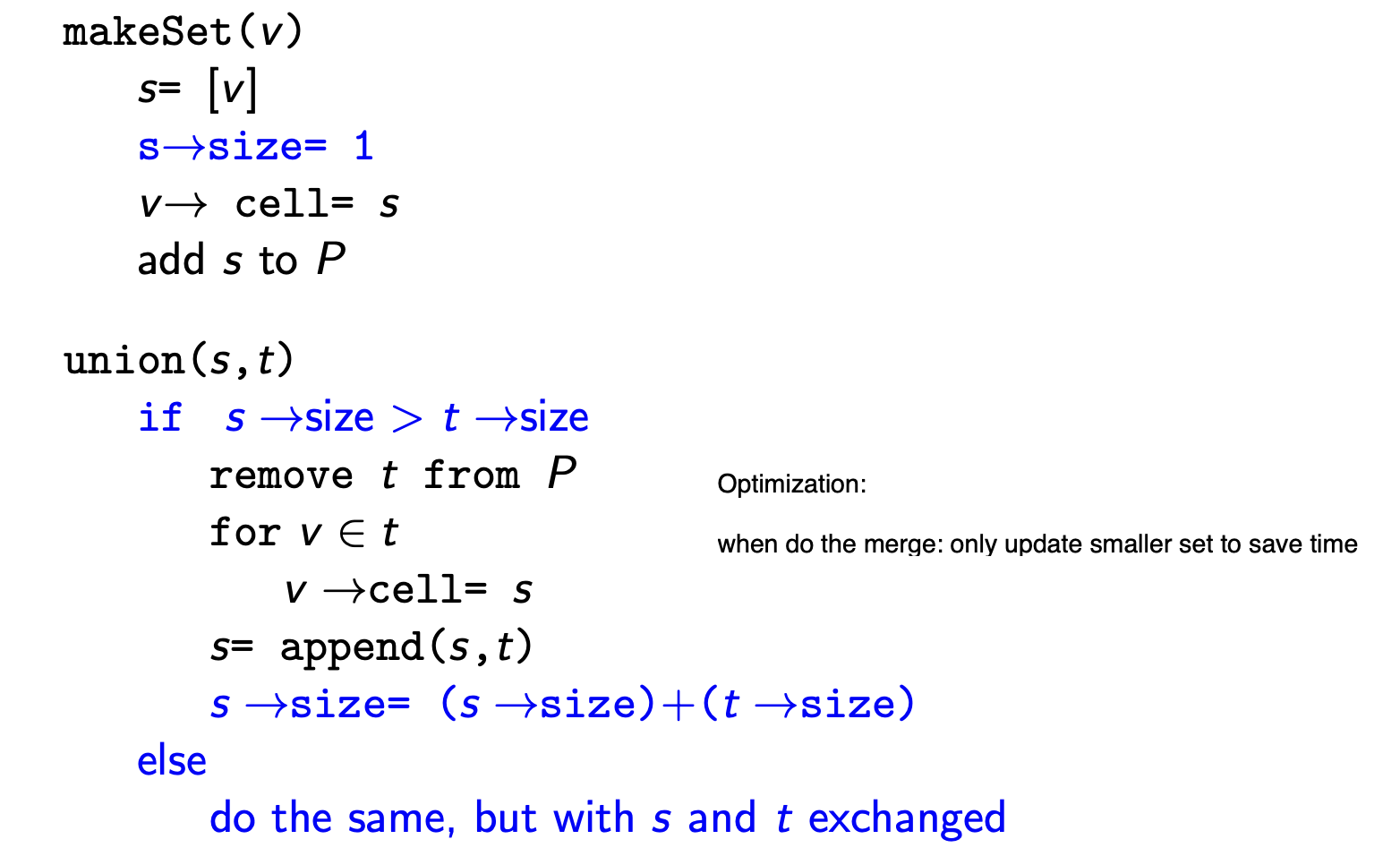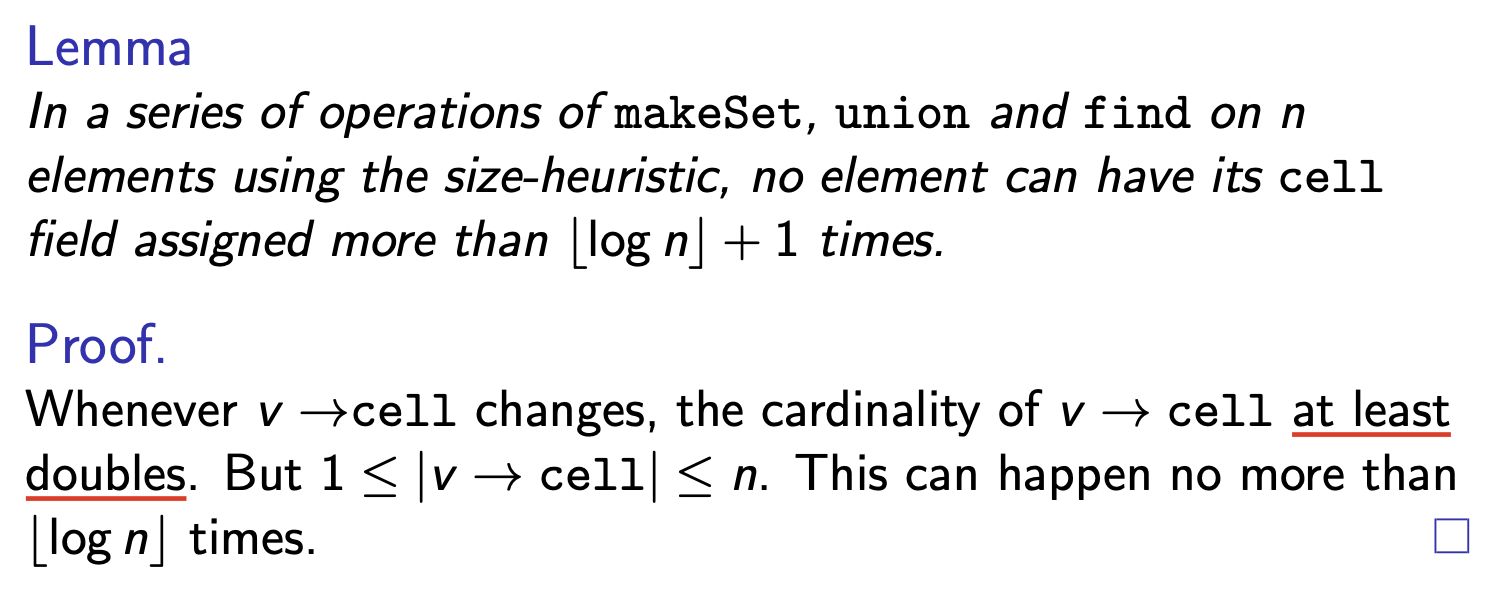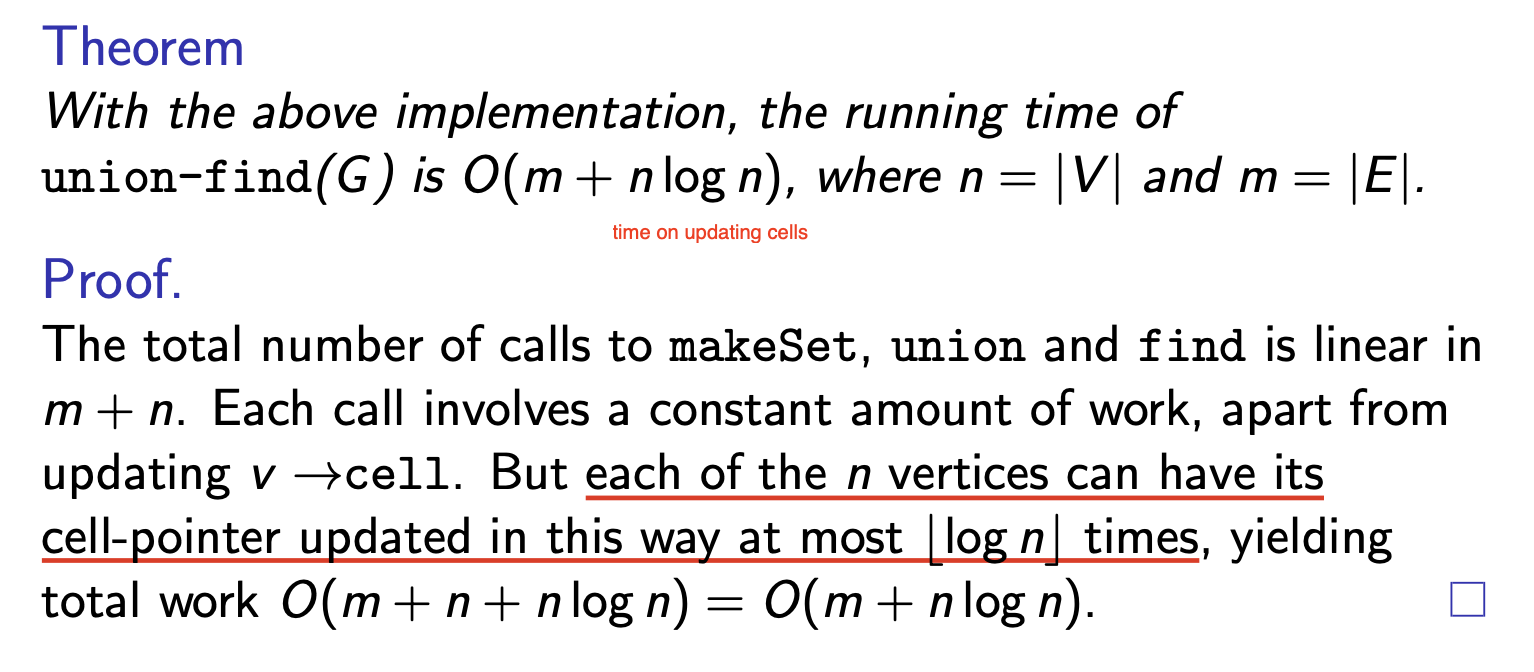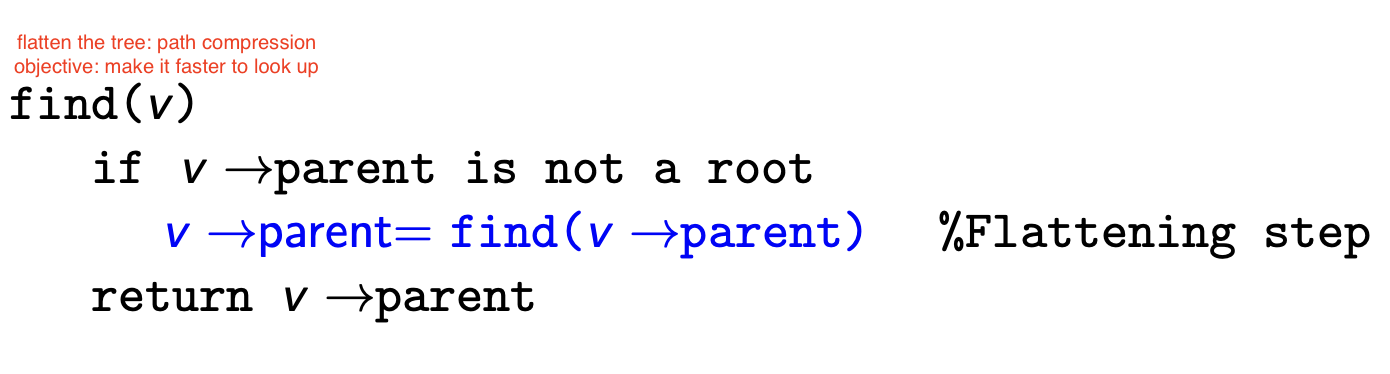# 高级算法设计:基础图算法

## Basic Graph Algorithms

Posted by R1NG on September 30, 2022 Viewed Times

# 图论算法初步

!!注: 完整详细的复习内容请参阅 《COMP36111 期末总复习》.

## 1. 有向图的基本概念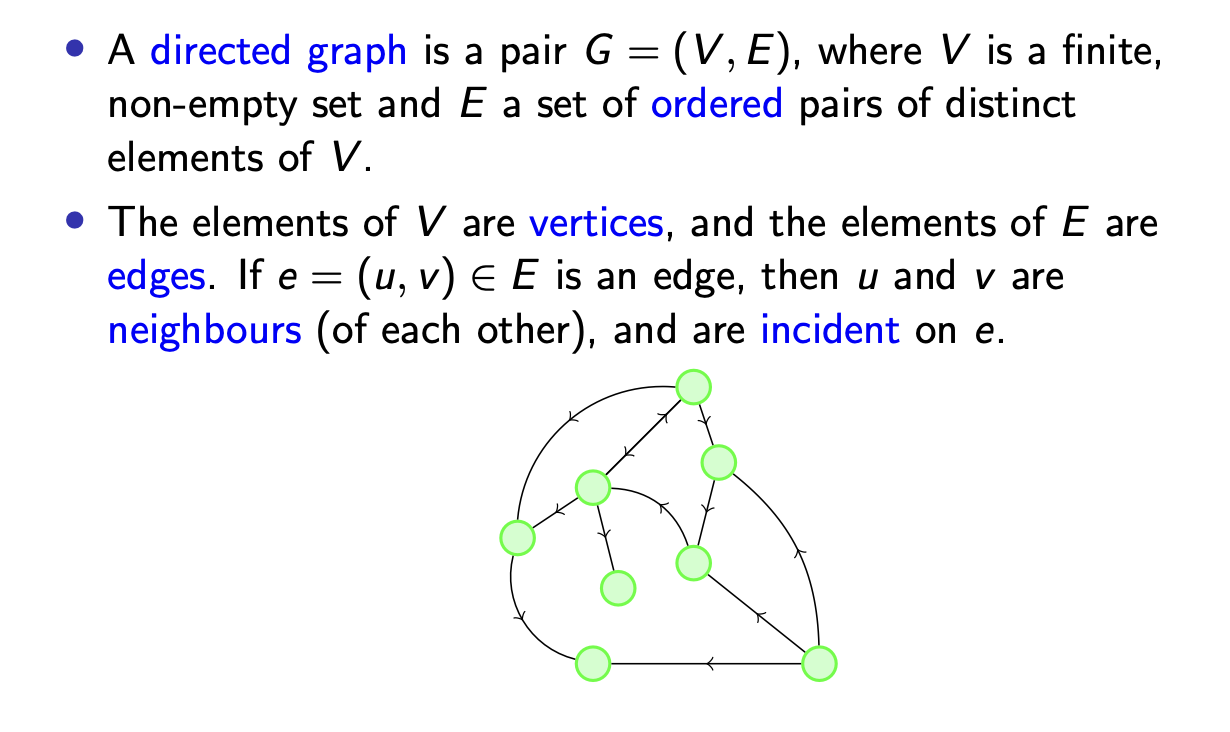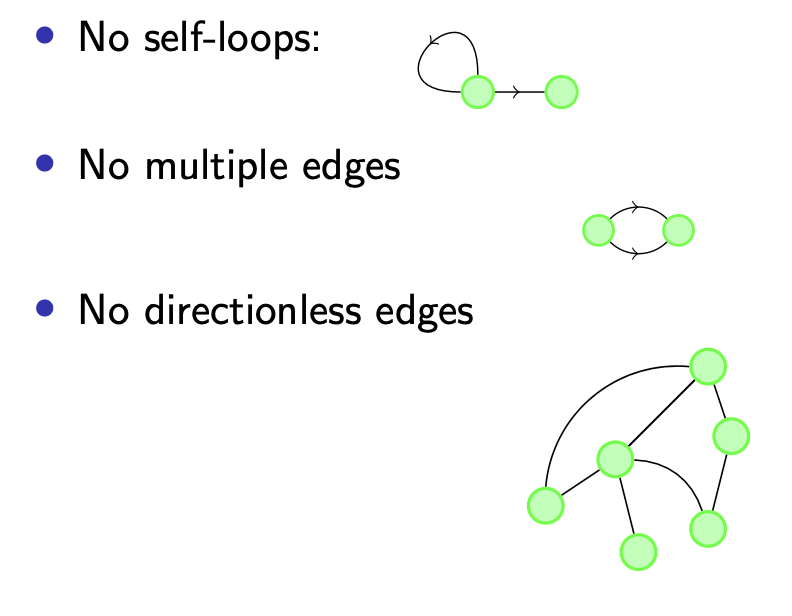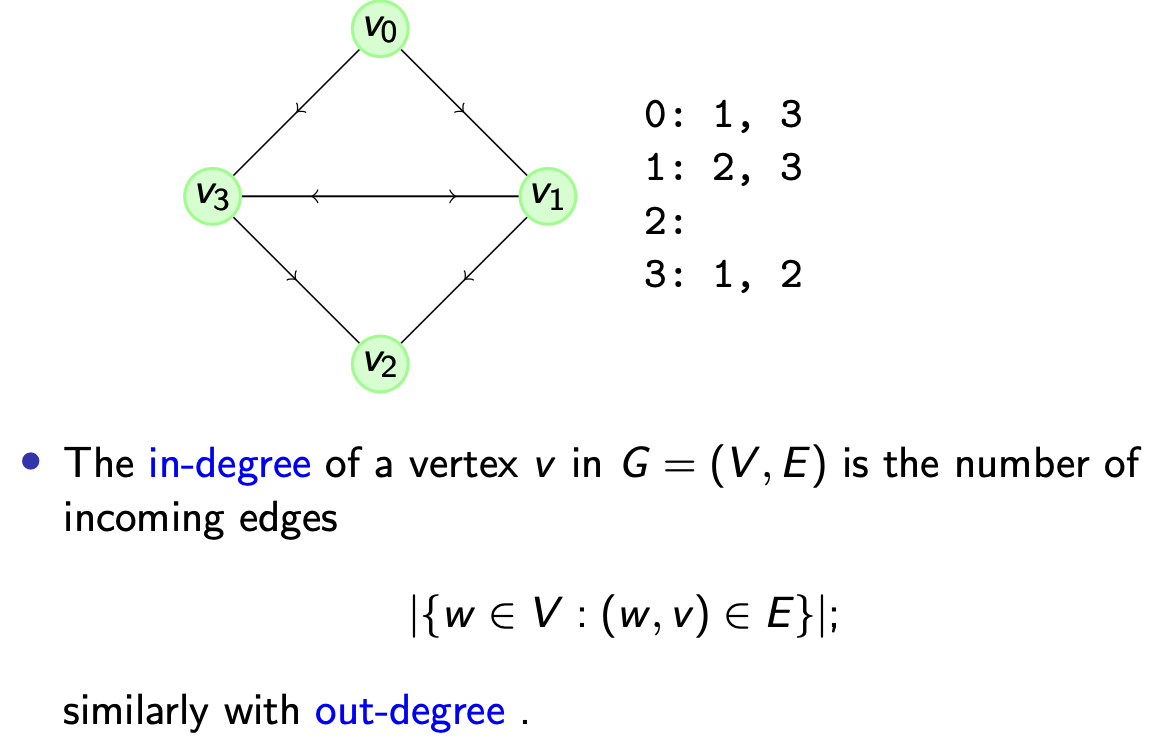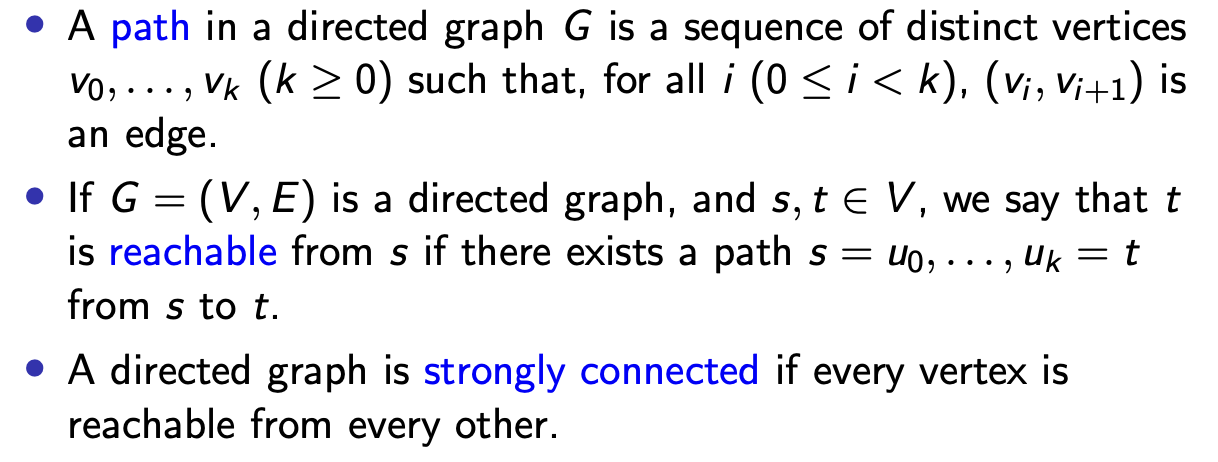1. st-CON:

给定某个有向图 $(V, E)$ 和图中的两个顶点 $s, t \in V$, 确定从 $s$ 到 $t$ 是否是 可达的. 它可以通过深度优先算法解决.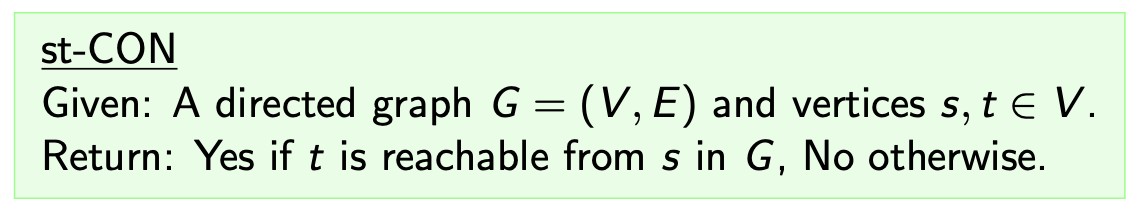2. STRONG CONNECTIVITY:

给定某个有向图 $(V, E)$, 确定它是否是强连通的.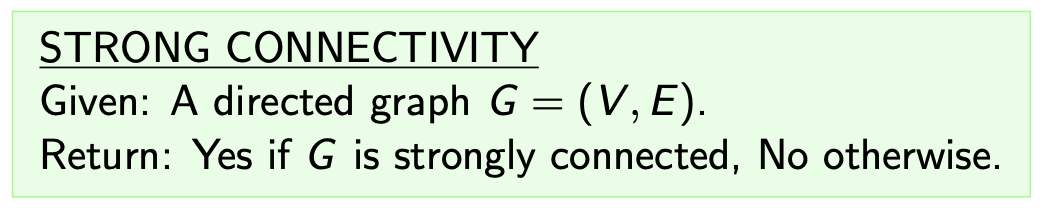## 2. 拓扑排序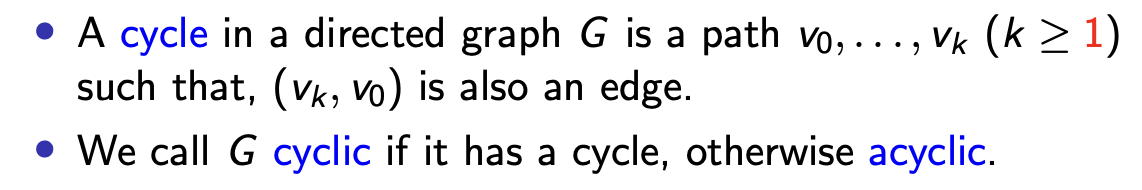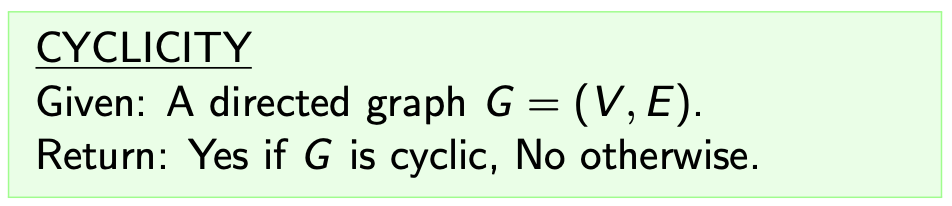$u_0, \cdots, u_{n-1}$

$(u_i, u_j)$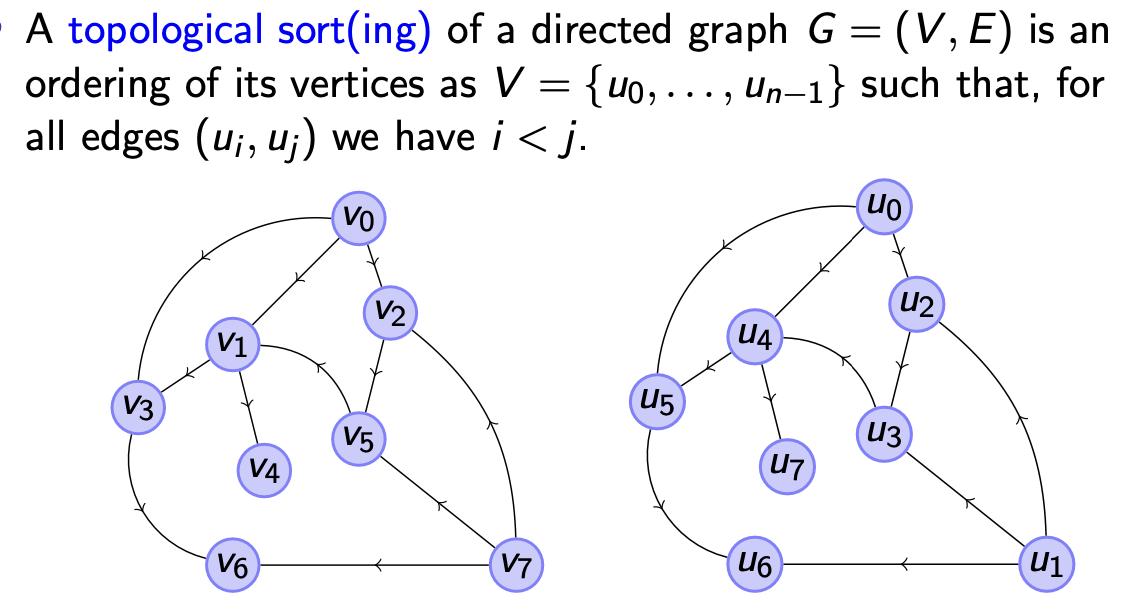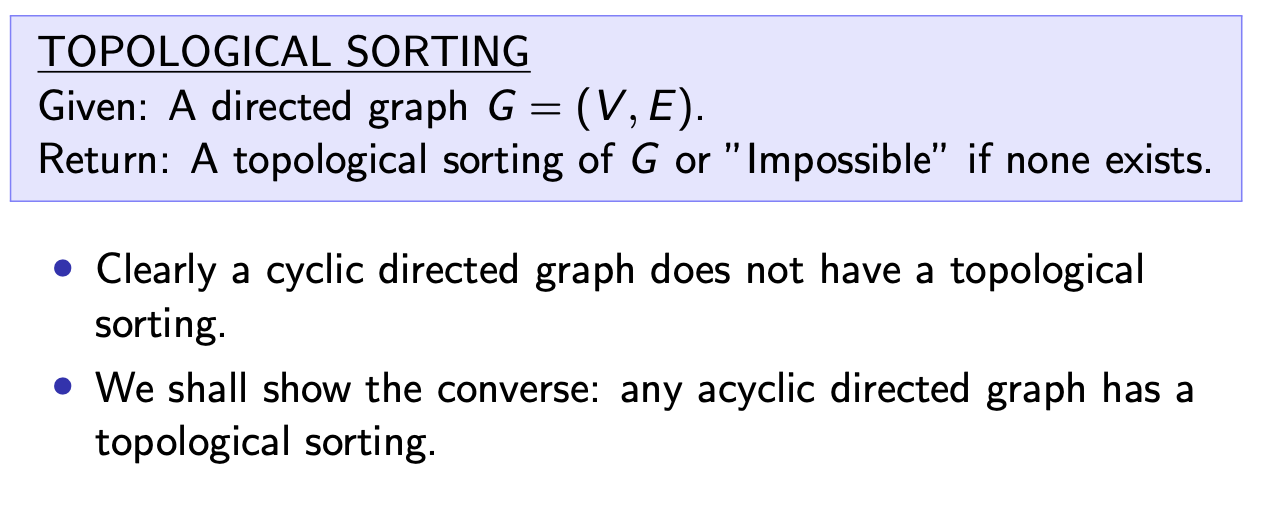1
2
3
4
5
6
7
8
9
10
11
12
13
14
15
16
17
18
19
20
21
22
23
24
25
26
27
28
29
30
31
32
33
34
def topSort(G):
# 计算图中每个顶点的入度
G.inDeg = handleIndegreeComputation(G)

# 栈 S 用于存储所有已发现但尚未使用深度优先遍历到的顶点
S = []

# i 在此处作为索引
i = 0

# sort 存储拓扑排序的最终结果
sort = [None for _ in range(len(G.vertices))]

# 使用深度优先搜索, 首先考虑所有起始点 (即入度为 0 的点)
for v in G.vertices:
if G.inDeg(v) == 0: S.push(v)

while len(S) != 0:
# 依次考虑栈首的点 (深度优先)
v = S.pop()
sort[i] = v
i += 1

# 遍历点 v 的每一个子节点, 在子节点的入度为 0 时再去遍历它, 确保排序的顺序性
for w in G.edges(v):
G.inDeg(w) -= 1
if G.inDeg(w) == 0:
S.push(w)

# 在全部遍历后, 若所有节点的入度都可变为 0 则说明该图是无环的
if i == n:
return sort
else:
return "Impossible"


1. 可以看出, 在任何一个顶点被从栈中弹出时, 它的所有前置节点必然已被弹出, 因此它们必然有更低的编号.
2. 若所有的顶点都被赋予某个编号, 则可确定我们必对这个有向图达成了拓扑排序.
3. 若某个顶点在最后被除去了编号, 则说明被检测的有向图 $G$ 必有一个 有向子图 $G’$, 其中 每个顶点的入度都不为 $0$. 因此可知 $G‘$ 和 $G$ 都是有环图.
4. 由此可知, 在此情况下对 $G$ 而言不存在拓扑排序. $\blacksquare$

$O(\vert V \vert + \vert E \vert).$

## 3. 有向图中的强连通分量问题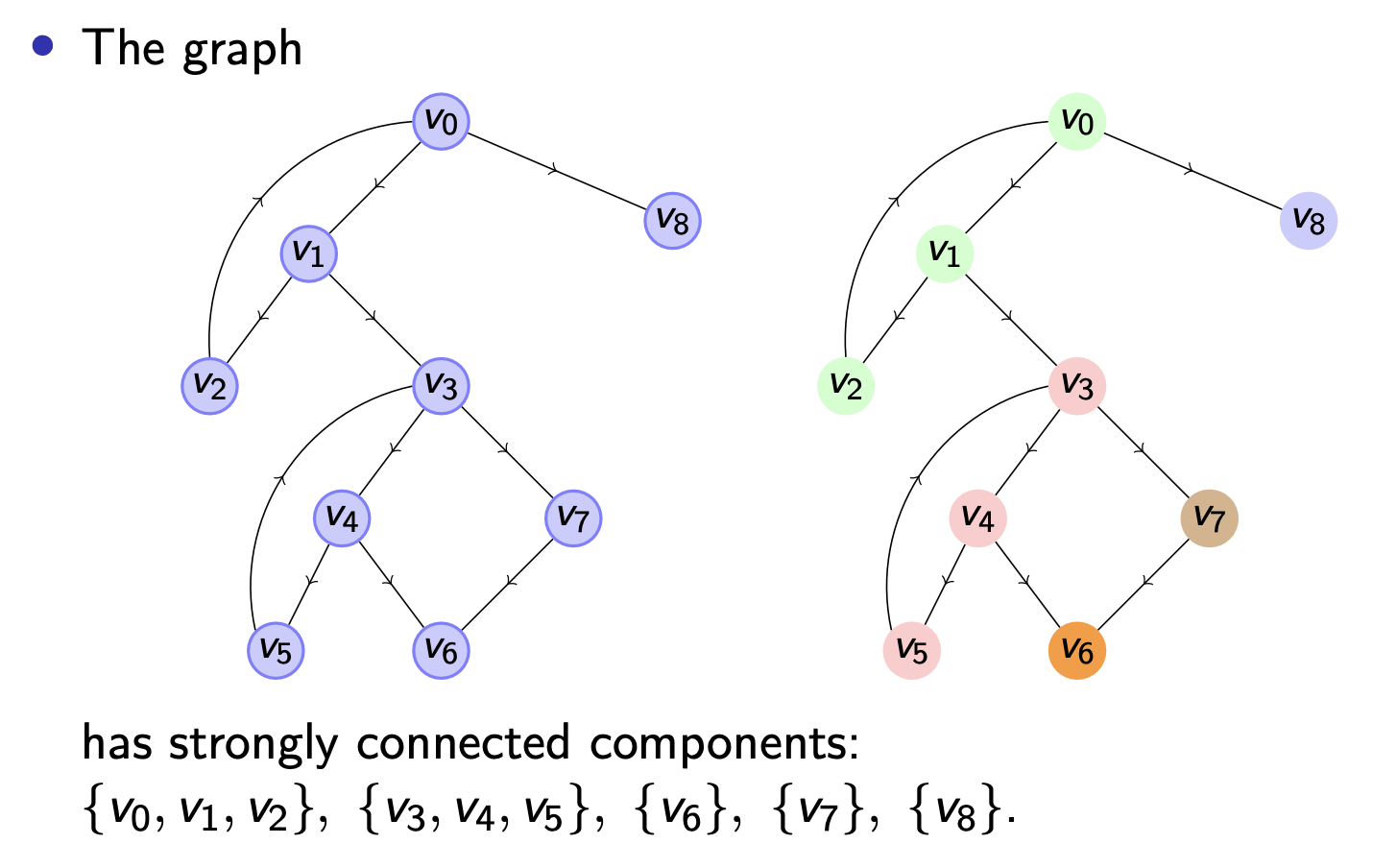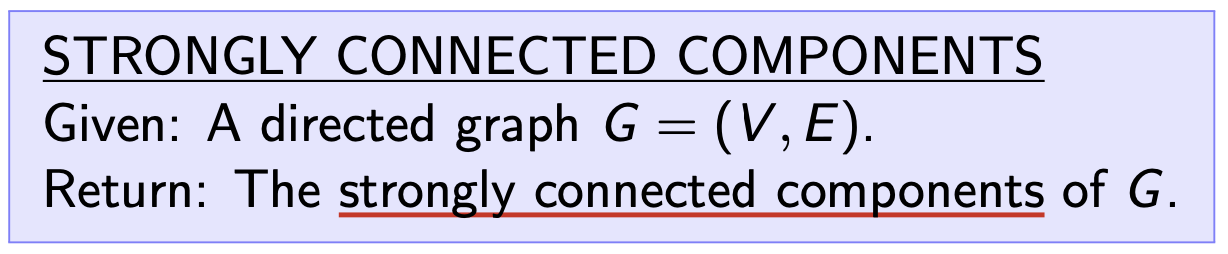### Kosaraju 算法

Kosaraju 算法的基本思路是:

1. 首先对给定有向图的 任意一个点 开始进行深度优先遍历, 然后依次将 完成遍历的节点存入某个栈中. 注意此处在遍历到每个节点时都会 先将这个点标记为 “visited”, 若该点的所有子节点都是 “visited”才算作完成遍历, 因此 被存入栈中的节点必属于某个强连通分量. 这一步 实际上完成的是对原图 $G$ 的反图 $H$ 的伪拓扑排序 $S$.

(为什么是 “伪拓扑排序”? 因为真正的拓扑排序在 有环图 上必不存在, 而任何强连通分量都必然是有环的. 所以这里的 “伪拓扑排序” 到底是什么? 它就是 将原来的强连通分量中环随机切掉一条边之后得到的无环图的拓扑排序.)

(为什么这条边是随机切掉的? 因为我们开始遍历时选择顶点的顺序是 随机 的. 因此在遍历强连通分量时, 最后遍历到的边也不是确定的. 根据算法逻辑可知, 切掉的边必然是 最后一个点到最初一个点之间连成的边.)

2. 由拓扑排序的定义可知, 它实际上已经将原图中所有的强连通分量归类排好序了 (考虑任意两个顶点 $v_i$, $v_j$, 可知要么有一条边 $(v_i, v_j)$, 也就是说它们同在一个强连通分量重, 要么这两个点互不隶属).
3. 由于对图的边取反 不会影响原来图中强连通分量之间的连通性, 而 原本不连通的分量之间在取反后还是不连通, 因此只需要对取反的图根据拓扑排序 $S$ 再执行一次深度优先搜索,就可以依次找出所有的强连通分量.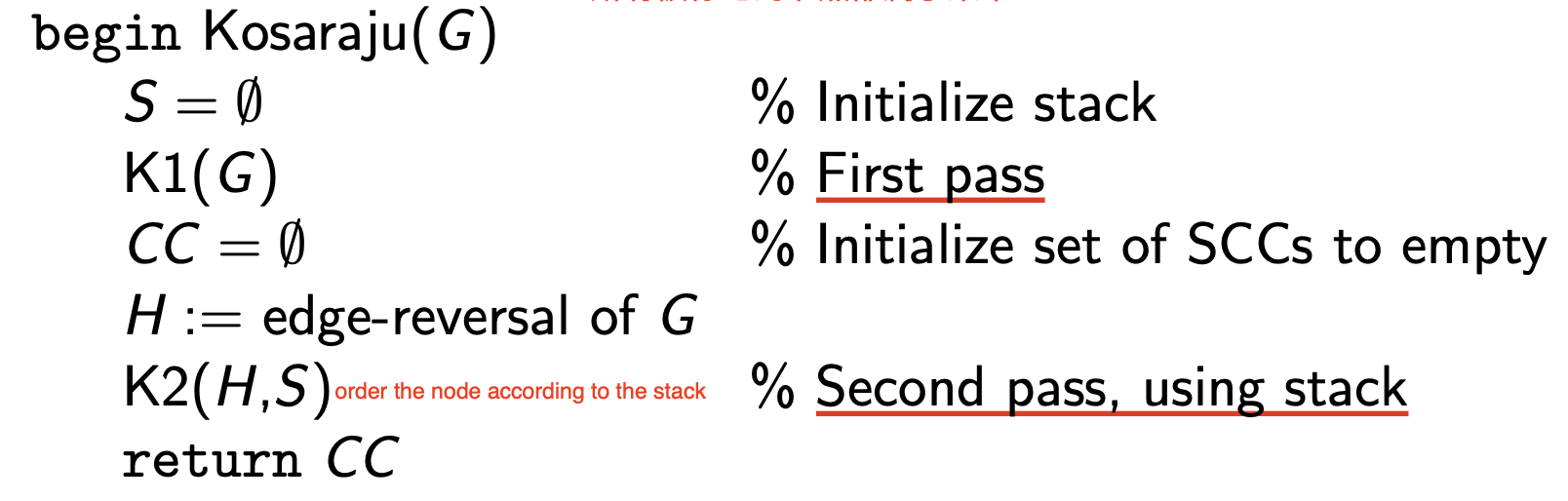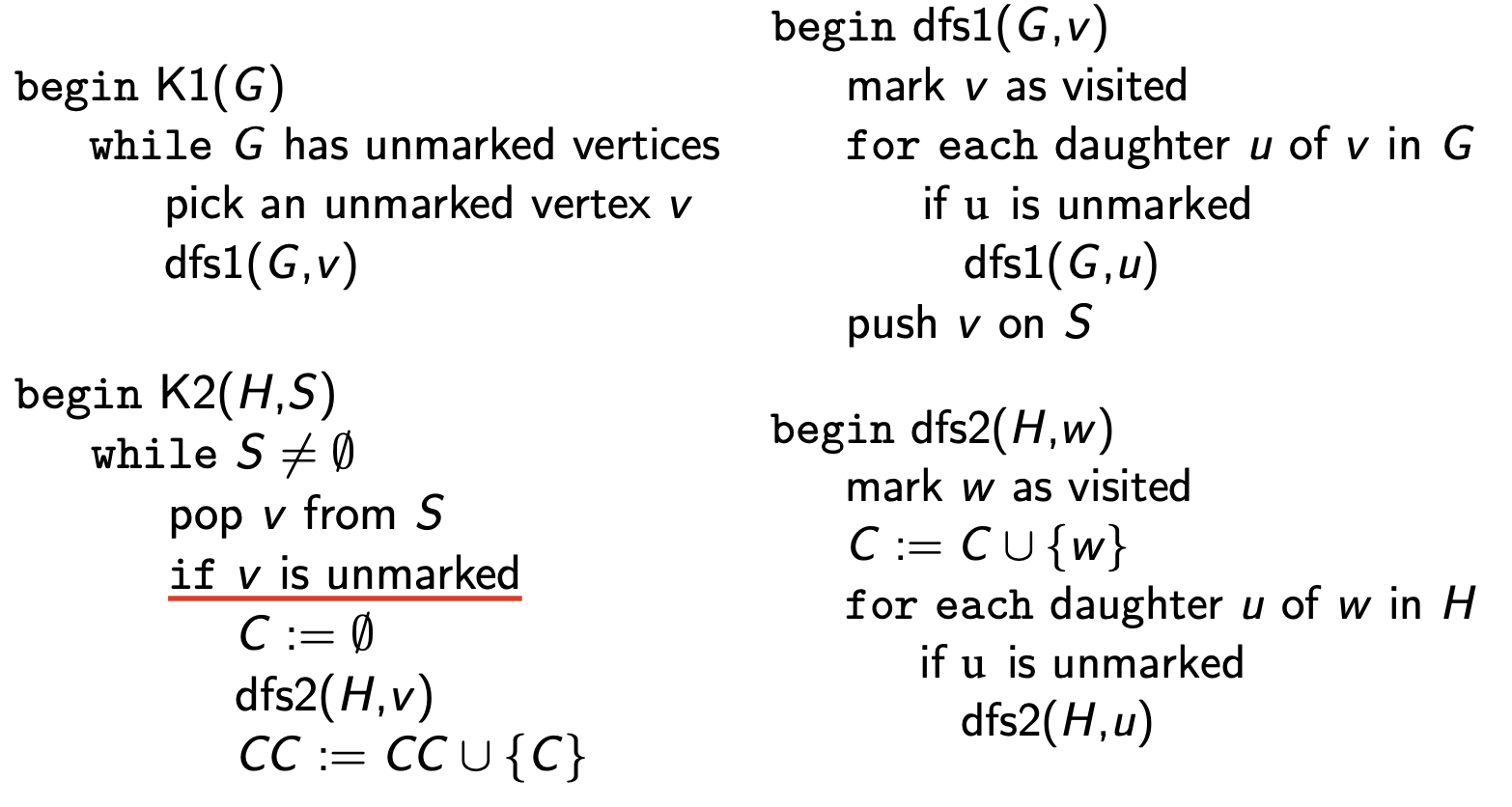1


### Tarjan 算法

Tarjan 算法是另一种基于深度优先搜索, 但 只需搜索一次 的算法: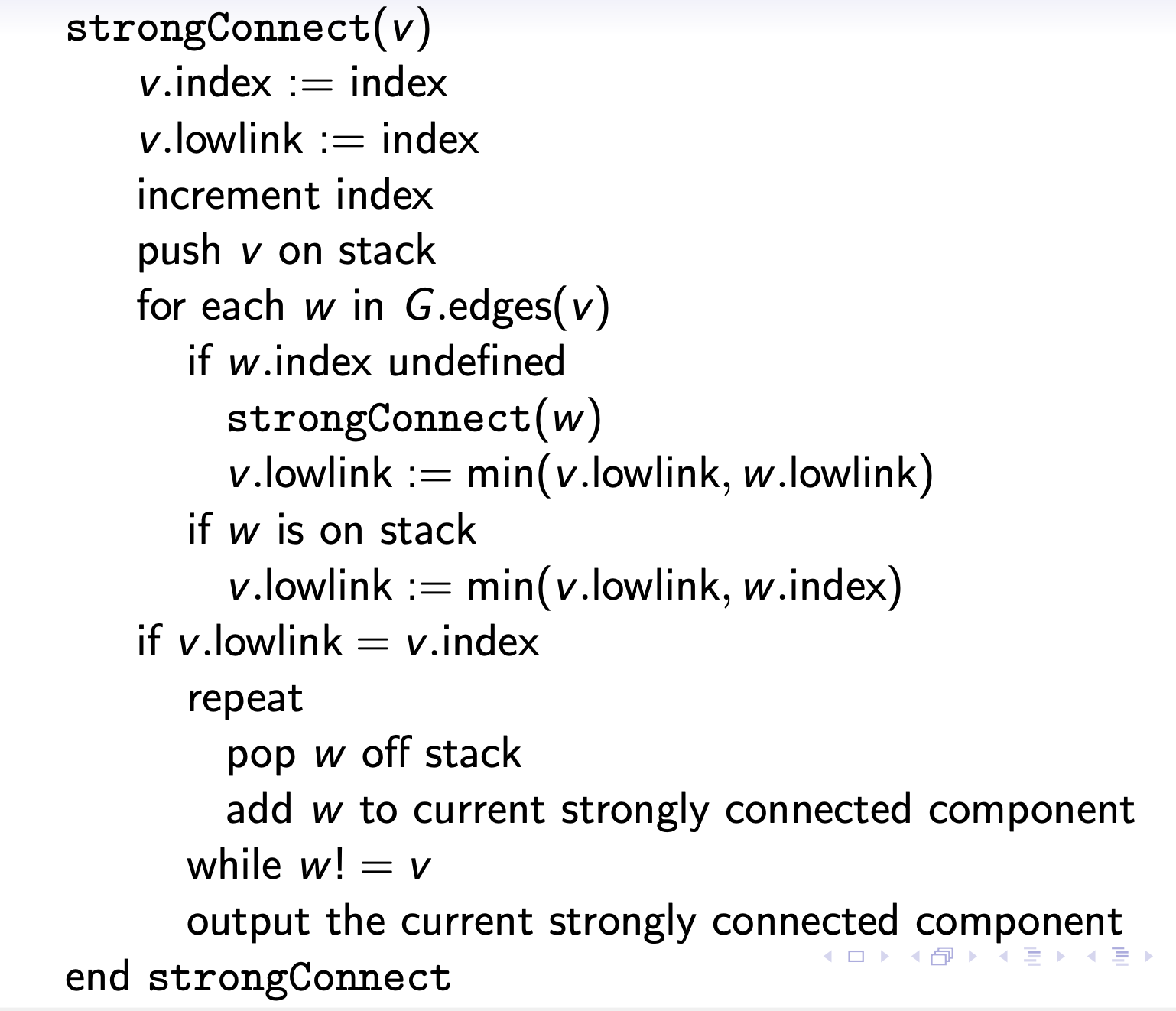## 4. 无向图的基本概念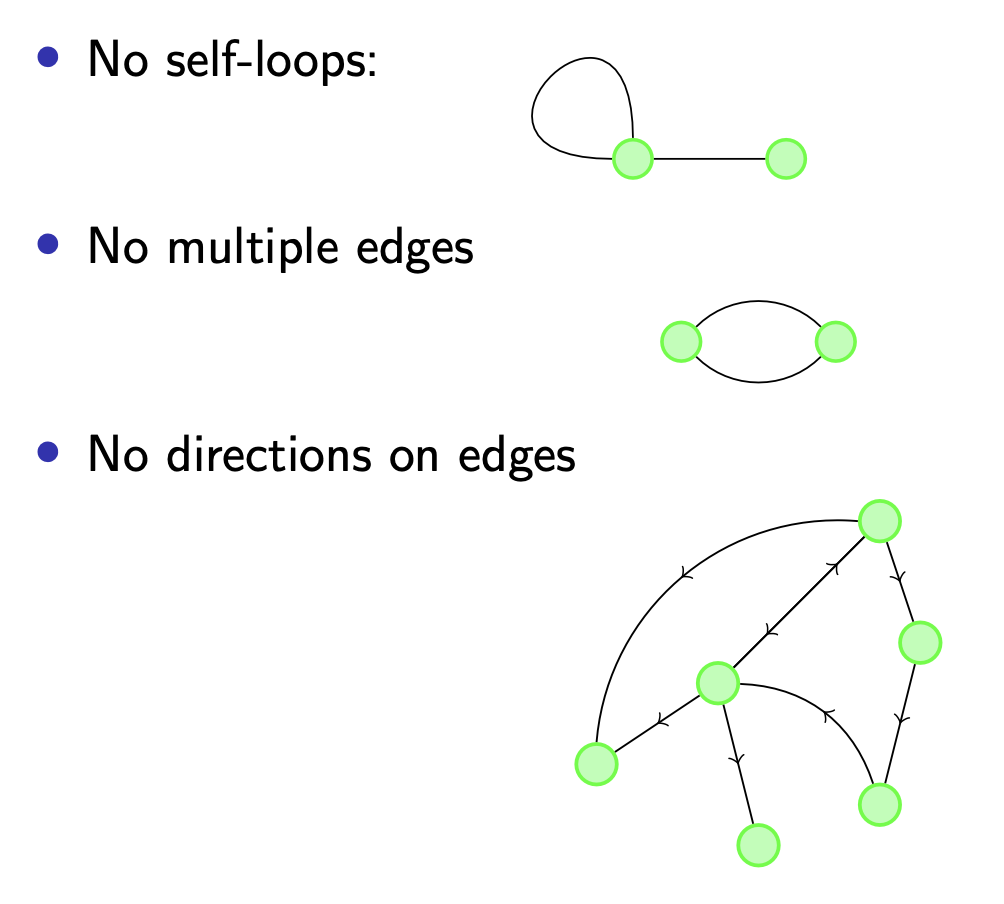## 5. 无向图的连通分量问题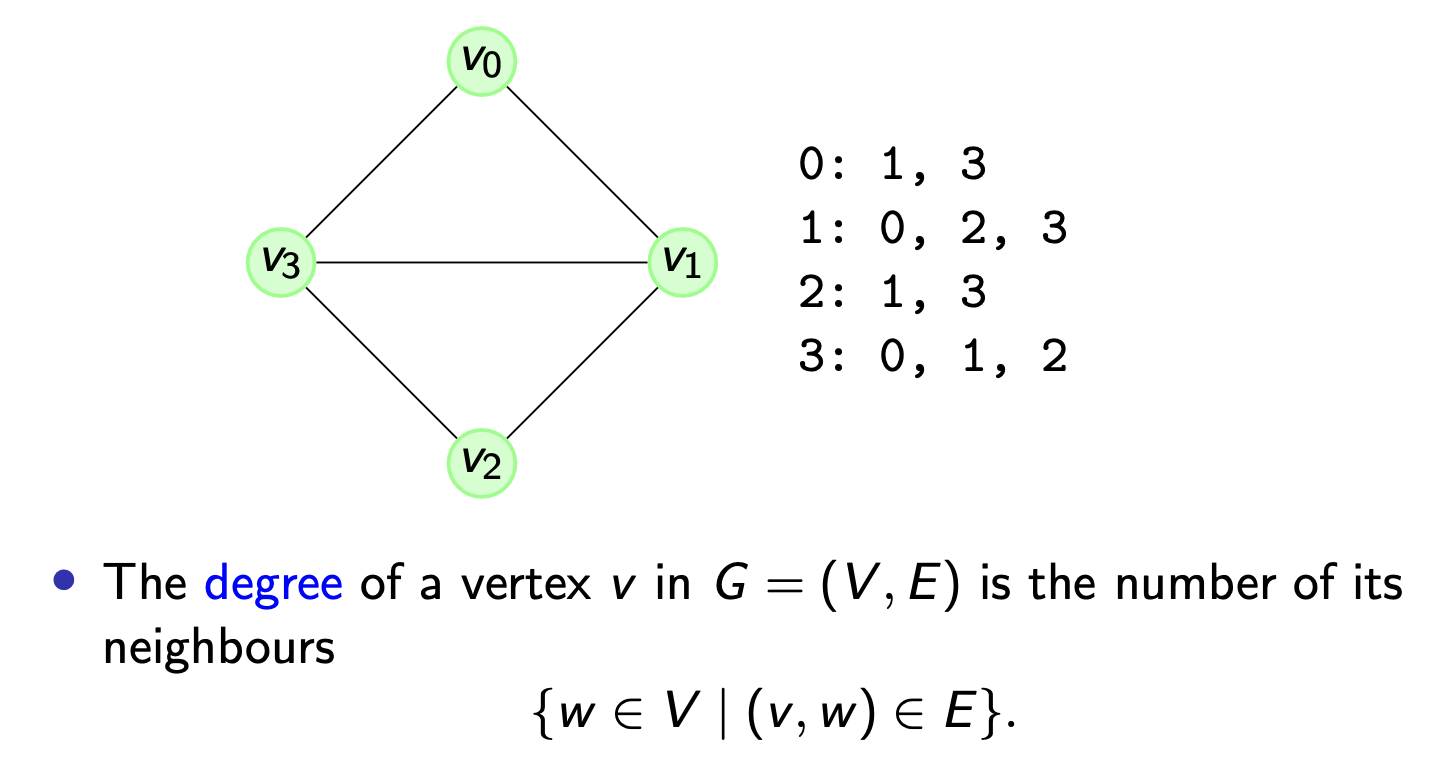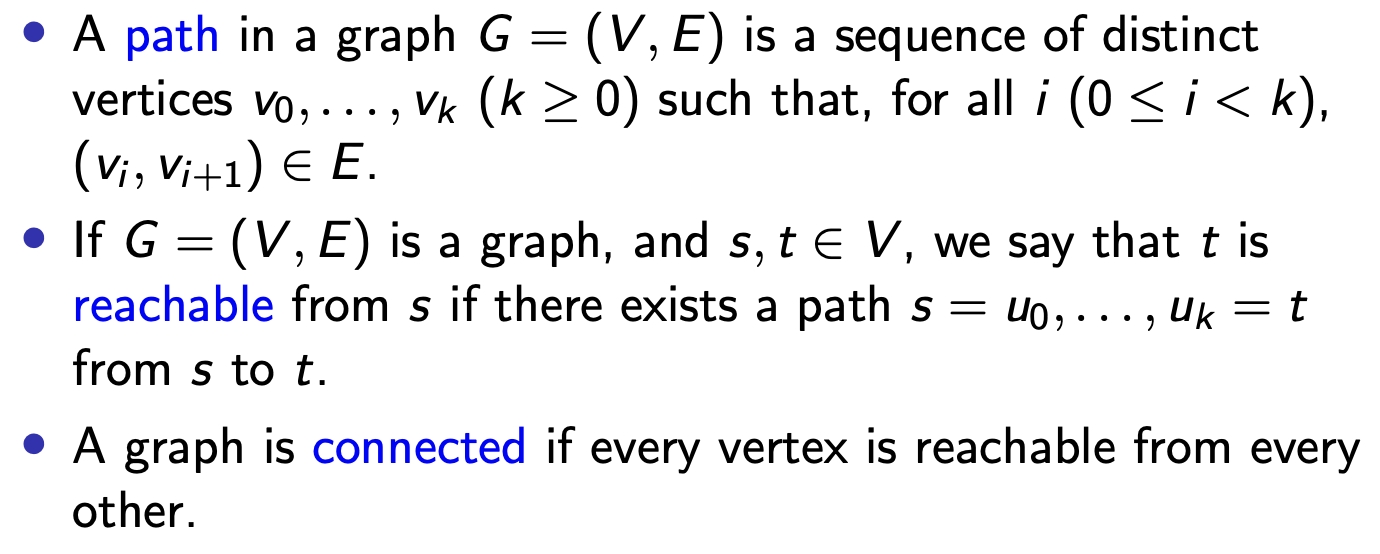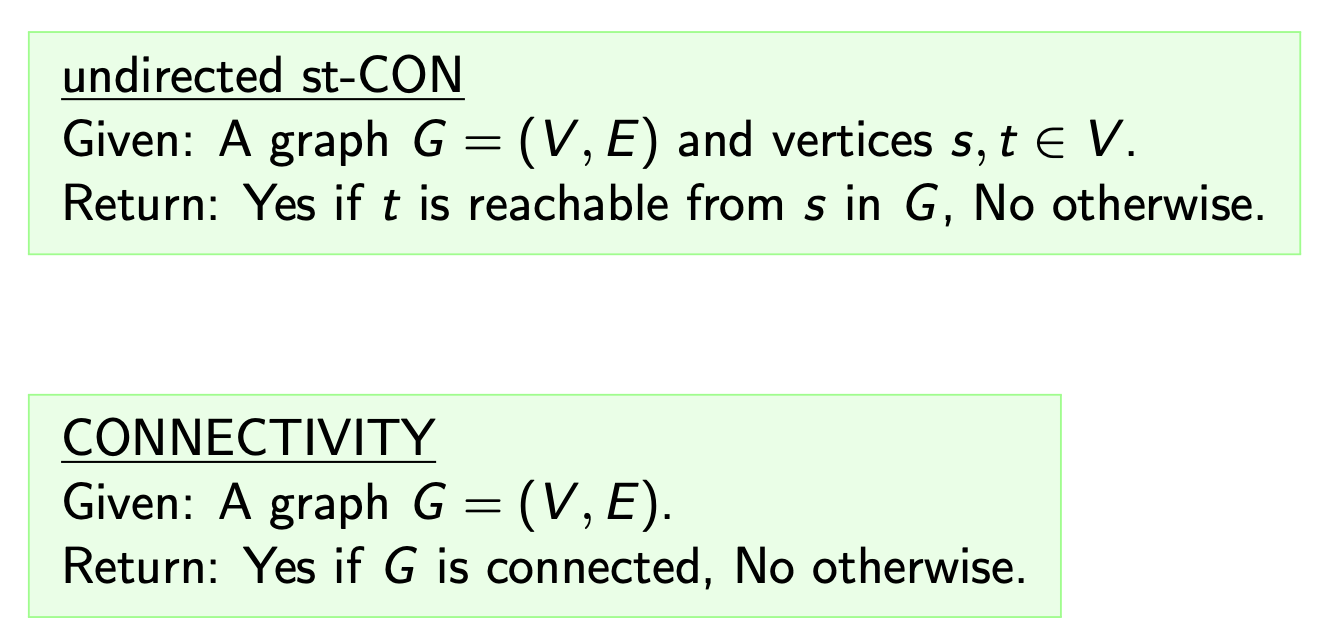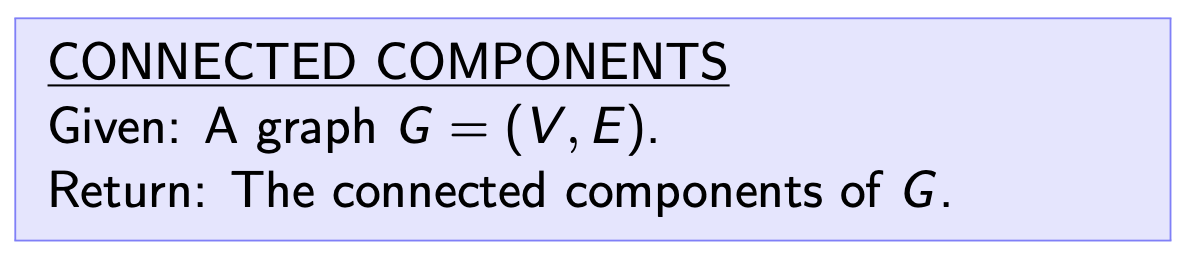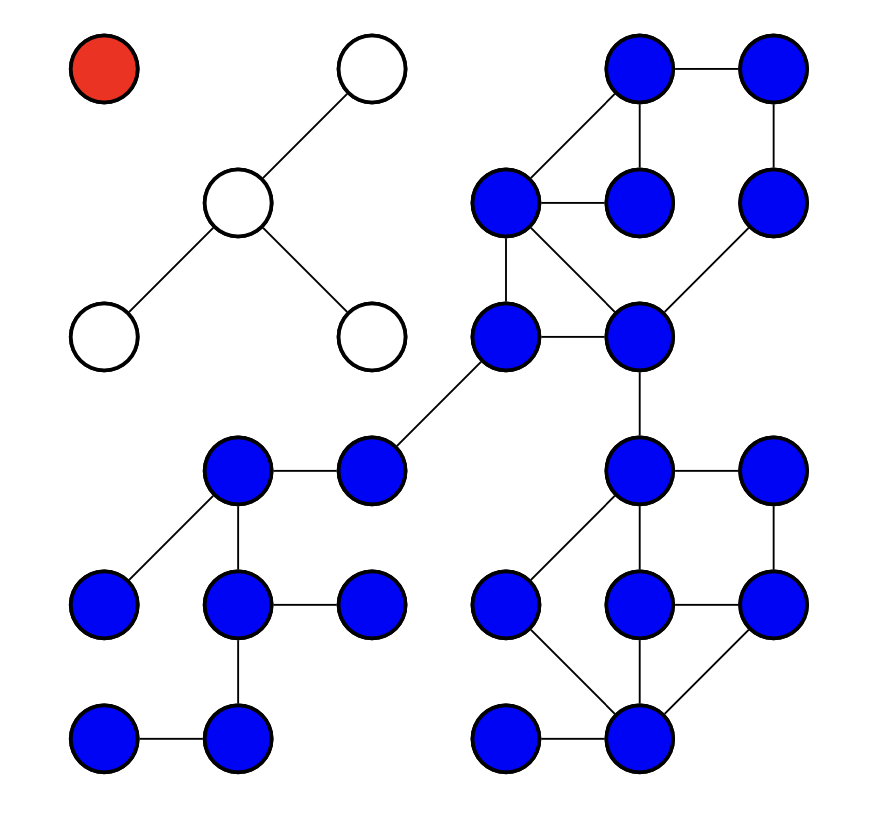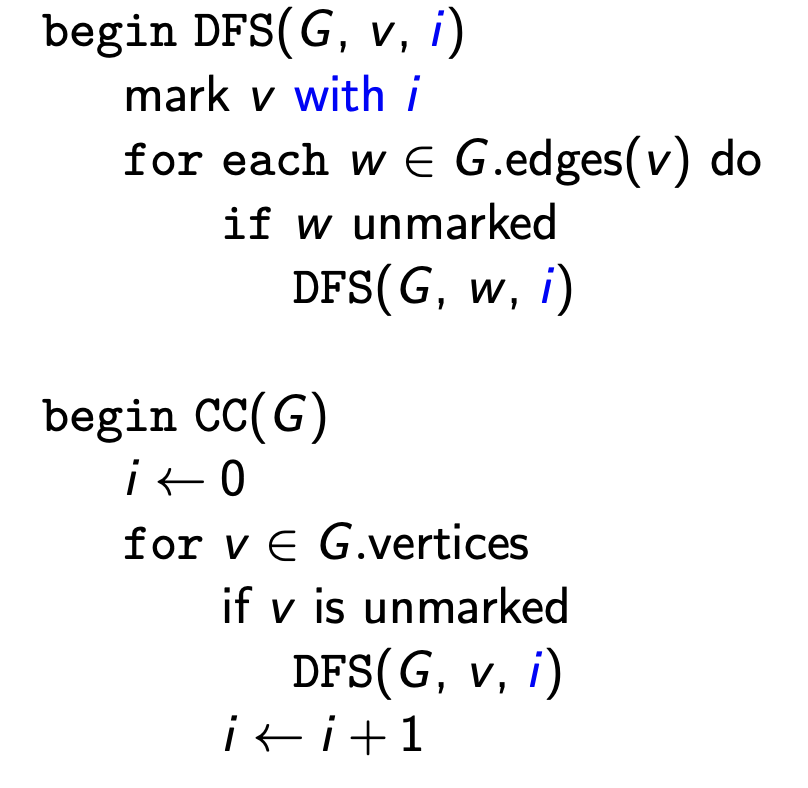## 6. Union-Find 结构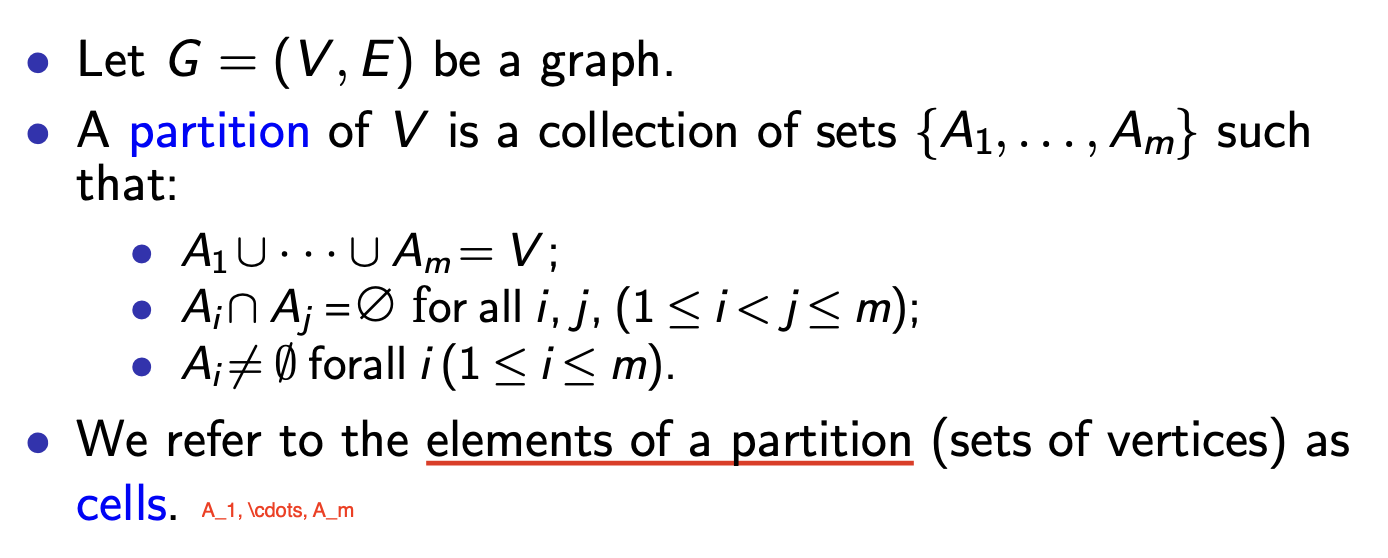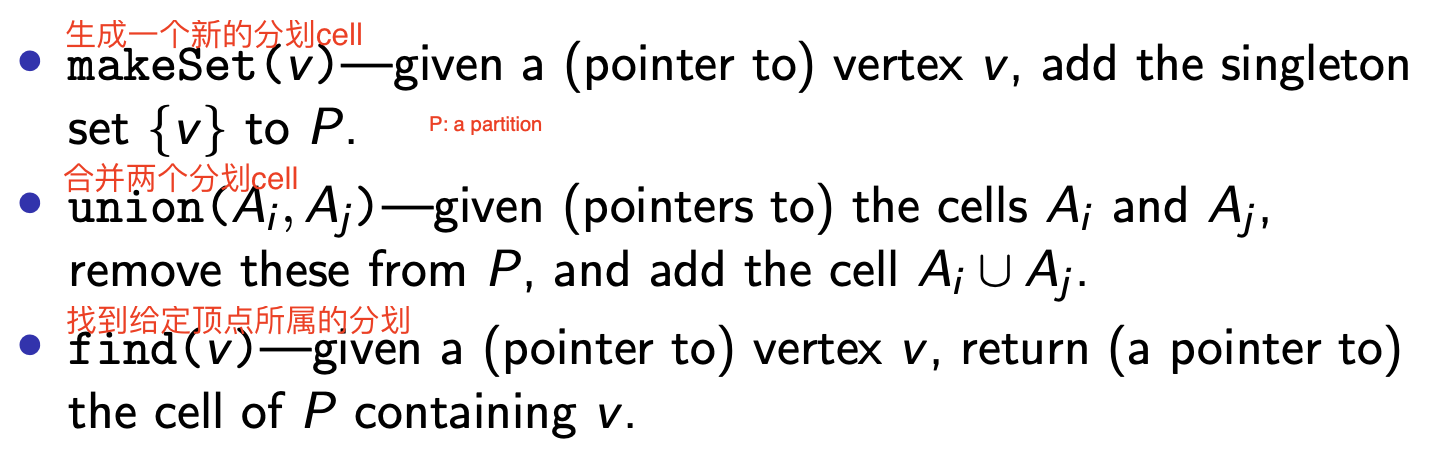1
2
3
4
5
6
7
8
9
10
11
12
13
def makeSet(v):
s = [v]
v.cell = s

def find(v):
return v.cell

def union(s, t):
P.remove(t)
s = append(s, t)    # this operation will also update p
for v in t:
v.cell = s